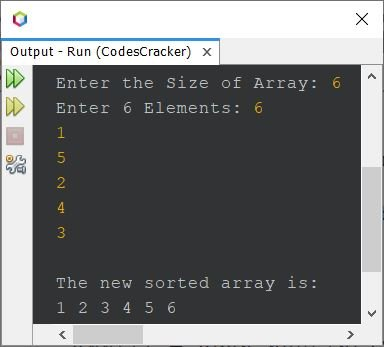# Insertion Sort Program in Java

This article covers a program in Java that sorts an array using insertion sort technique. In both the program given below, the size and array both are received by user at run-time of the program.

Note - If you're not aware about, how the insertion sort performs, then refer to Insertion Sort Algorithm and Example. Now let's create the program.

## Insertion Sort in Java using for Loop

The question is, write a Java program to perform insertion sort based on an array of n elements. The program given below is its answer. This program is created without using function.

```import java.util.Scanner;

public class CodesCracker
{
public static void main(String[] args)
{
int n, i, j, element;
Scanner scan = new Scanner(System.in);

System.out.print("Enter the Size of Array: ");
n = scan.nextInt();
int[] arr = new int[n];
System.out.print("Enter " +n+ " Elements: ");
for(i=0; i<n; i++)
arr[i] = scan.nextInt();

for(i=1; i<n; i++)
{
element = arr[i];

for(j=(i-1); j>=0 && arr[j]>element; j--)
arr[j+1] = arr[j];

arr[j+1] = element;
}

System.out.println("\nThe new sorted array is: ");
for(i=0; i<n; i++)
System.out.print(arr[i]+ " ");
}
}```

The snapshot given below shows the sample run of above program with user input 6 as size and 6, 1, 5, 2, 4, 3 as six elements:## Insertion Sort in Java using while Loop

This is the same program as of previous, but created using while loop, instead of for.

```import java.util.Scanner;

public class CodesCracker
{
public static void main(String[] args)
{
int n, i, j, element;
Scanner scan = new Scanner(System.in);

System.out.print("Enter the Size of Array: ");
n = scan.nextInt();
int[] arr = new int[n];
System.out.print("Enter " +n+ " Elements: ");
i=0;
while(i<n)
{
arr[i] = scan.nextInt();
i++;
}

i=1;
while(i<n)
{
element = arr[i];

j=i-1;
while(j>=0 && arr[j]>element)
{
arr[j+1] = arr[j];
j--;
}

arr[j+1] = element;
i++;
}

System.out.println("\nThe new sorted array is: ");
i=0;
while(i<n)
{
System.out.print(arr[i]+ " ");
i++;
}
}
}```

#### Same Program in Other Languages

Java Online Test

« Previous Program Next Program »